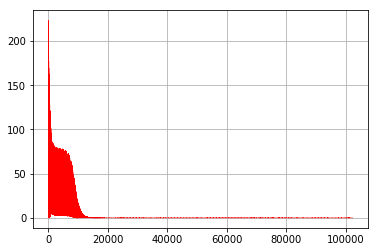2 views

I am trying to use a multi-layer neural network to predict nth square.

I have the following training data containing the first 99 squares

1 1 2 4 3 9 4 16 5 25 ... 98 9604 99 9801

This is the code:

import numpy as np

import neurolab as nl

text = np.loadtxt('data_sq.txt') # Separate it into datapoints and labels

data = text[:, :1]

labels = text[:, 1:] # Define a multilayer neural network with 2 hidden layers; # First hidden layer consists of 10 neurons

# Second hidden layer consists of 6 neurons

# Output layer consists of 1 neuron nn = nl.net.newff([[0, 99]], [10, 6, 1])

# Train the neural network error_progress = nn.train(data, labels, epochs=2000, show=10, goal=0.01)

# Run the classifier on test datapoints print('\nTest results:')

data_test = [, ]

for item in data_test:

print(item, '-->', nn.sim([item]))

Which prints 1 for both 100th and 101st squares:

Test results:  --> [ 1.]  --> [ 1.]

What is the right way to do this?

by (108k points)

You do not need a predictive model in the deterministic case, such as when

y=x*X  is known.

If it must really be done, it can be done in TensorFlow, like so:

# input placeholder

_x = tf.placeholder(dtype=tf.float32)

# a non-linear activation of the form y = x^2

_y = tf.square(_x

)

#

draw 5 samples from a distribution

in_x = np.random.uniform(0, 100,

5)

s

ess = tf.Session(

)

wit

h sess.as_default():

for x in in_x:

print(sess.run(_y, feed_dict={_x: x}))

It is not a neural network because you already know the function map.

Now, say, that you didn’t. Assume that the following data was available to us but without any prior knowledge of the relationship:

# 1024 integers randomly sampled from the range 1-5

x_train = np.random.randint(1, 5, 1024)

y_train = np.square(x_train)

Then, we could write a network (I’ll do it with one hidden layer), like so:

n_epochs = 100

n_neurons = 128

tf.reset_default_graph()

sess = tf.Session()

with sess.as_default()

x = tf.placeholder(dtype=tf.float32)

y = tf.placeholder(dtype=tf.float32)

# Hidden layer with 128 neurons

w1 = tf.Variable(tf.truncated_normal([1, n_neurons], stddev=0.1))

b1 = tf.Variable(tf.constant(1.0, shape=[n_neurons]))

# This is not a standard activation

# Output layer

w2 = tf.Variable(tf.truncated_normal([n_neurons, 1], stddev=0.1))

b2 = tf.Variable(tf.constant(1.0))

loss = tf.losses.mean_squared_error(labels=y, predictions=prediction)

global_step = tf.Variable(0, trainable=False)

sess.run(tf.global_variables_initializer())

for epoch in range(n_epochs):

for idx, x_batch in enumerate(x_train):

y_batch = y_train[idx]

_, step, _pred, _loss = sess.run([train_op, global_step, prediction, loss], feed_dict={x: x_batch, y: y_batch})

print ("Step: {}, Loss: {}, Value: {}, Prediction: {}".format(_step, _loss, y_batch, int(_pred)))

The model converges at around 16 epochs as is seen in the loss plot below.Although the test accuracy is still way off at 50%, we observe that the model has, in fact, learned a pretty good approximation of the function. Further, even for a previously unseen example, the model is able to make a reasonable prediction:

test_pred = sess.run(prediction, feed_dict={x: 6})

print (test_pred)

# output: 33.570126

For x = 6, it predicts y = 33.57.